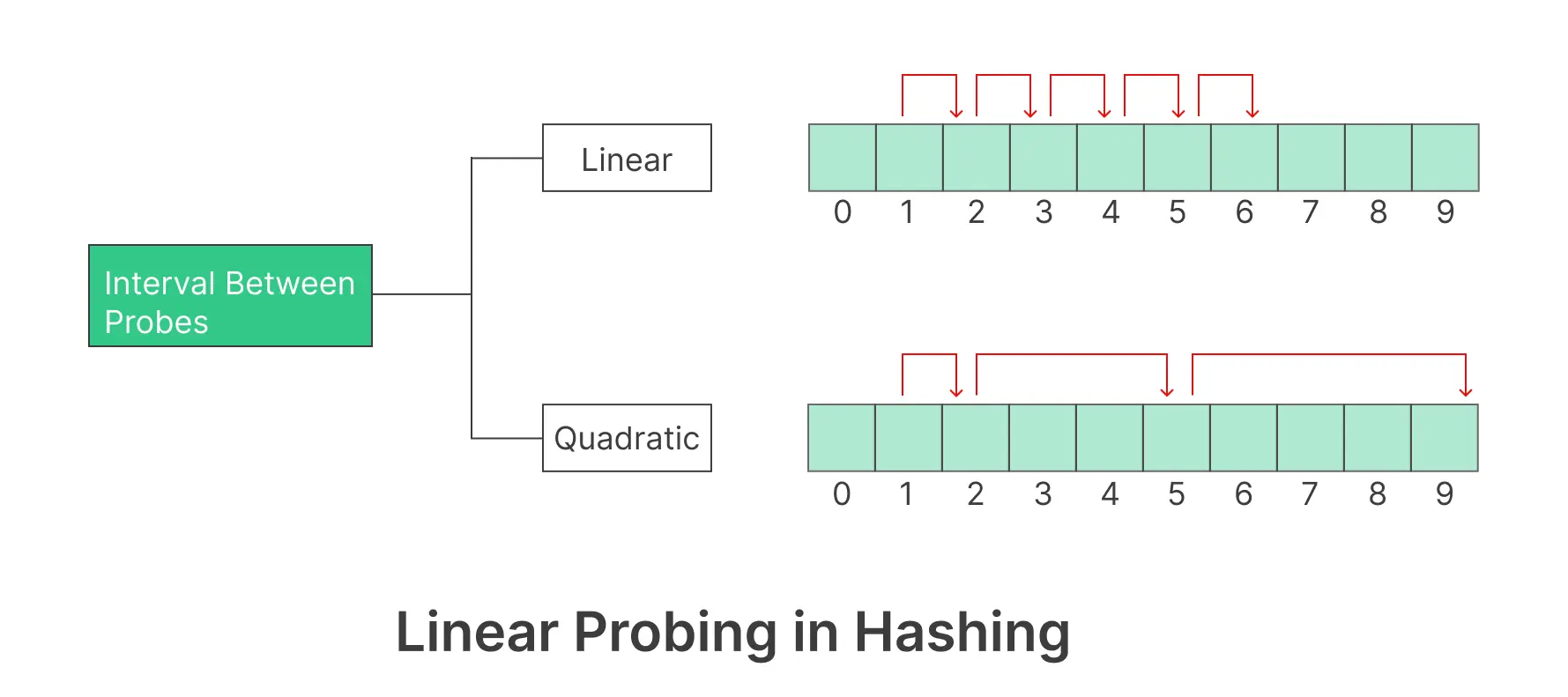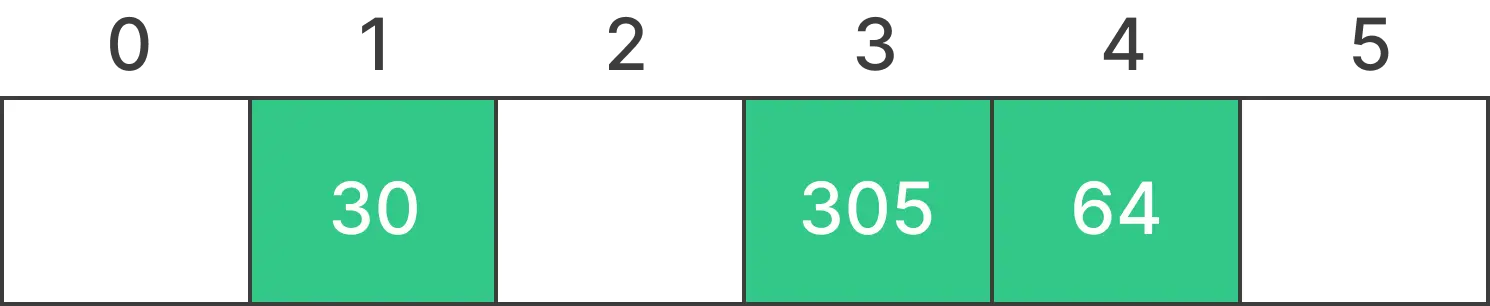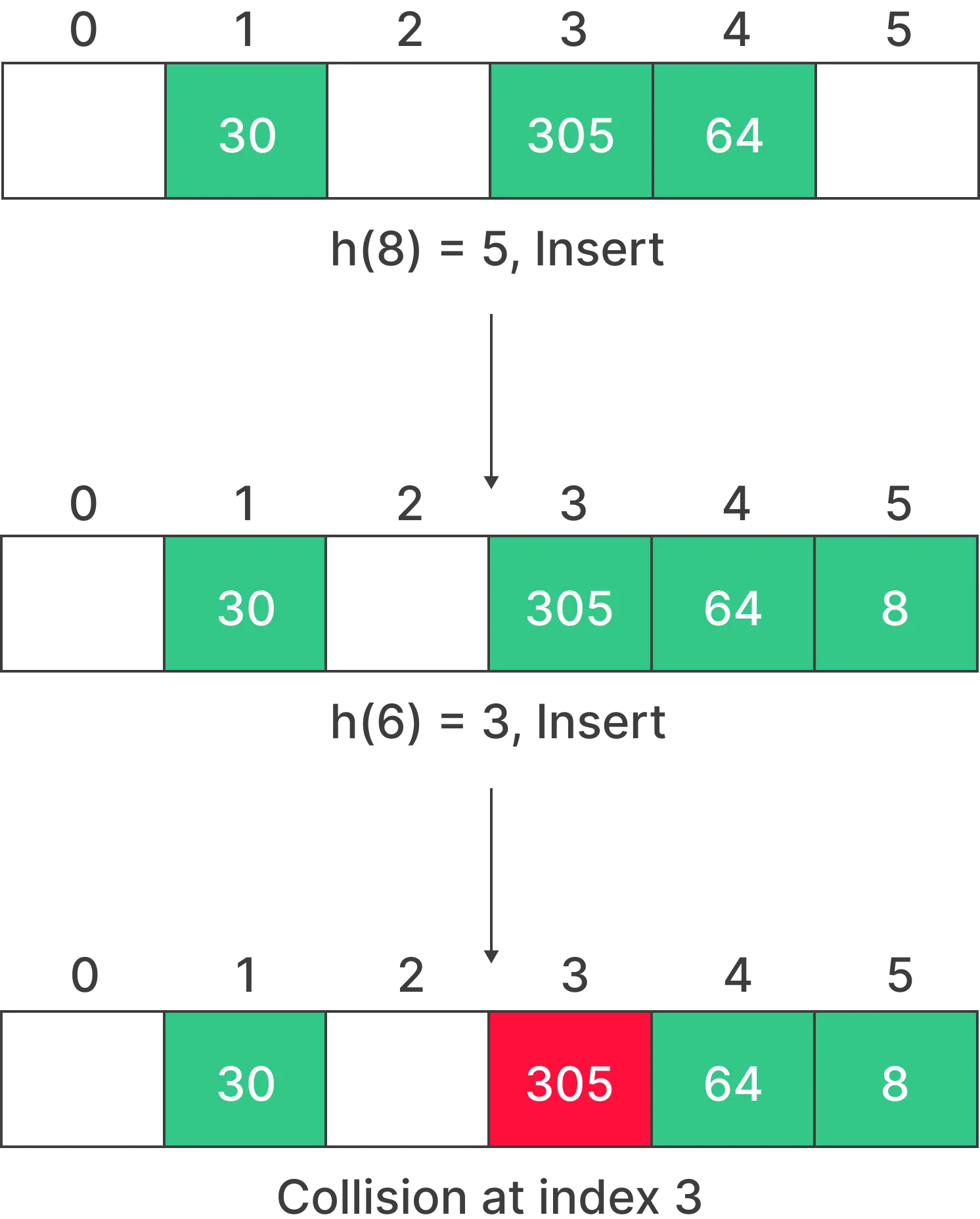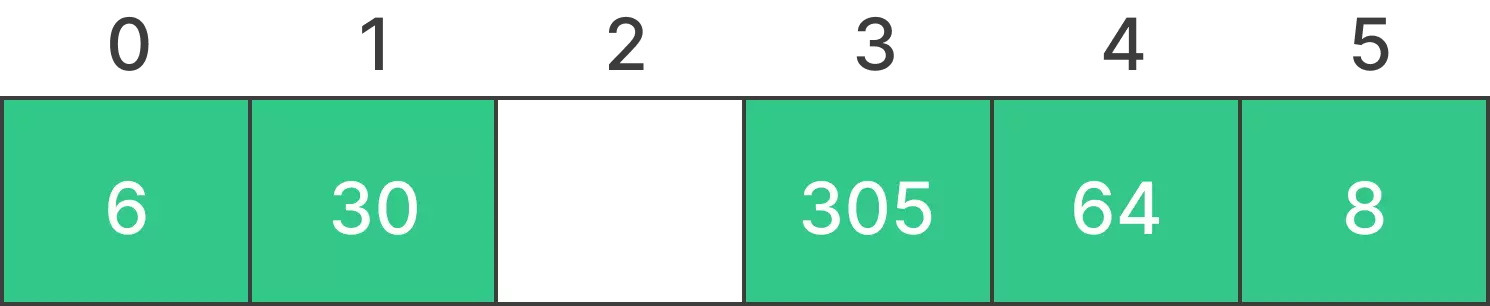# Linear Probing in Hashing## Introduction to Linear Probing in Hashing

In the realm of data structures and algorithms, one of the fundamental concepts is linear probing in hash tables. This technique allows for efficient storage and retrieval of data by handling collisions gracefully.

Let’s jump into the article to know more about Linear Probing in Hashing and also you will get to know about the explaining its inner workings and applications.

## Understanding Linear Probing in Hashing

Linear probing in Hashing is a collision resolution method used in hash tables. Collisions occur when two keys produce the same hash value, attempting to map to the same array index. Linear probing deals with these collisions by searching for the next available slot linearly in the array until an empty slot is found.

## Linear Probing in Hashing in Python### Working of Linear Probing in Hashing

• Calculate the initial hash value for the key.
• If the slot is empty, place the key-value pair there.
• If the slot is occupied, probe linearly by moving to the next slot.
• Repeat steps 2 and 3 until an empty slot is found, and insert the key-value pair there.

### Linear Probing in Hashing Example

Suppose a hash length of 6  and we want to insert the elements of A.

• A = {30,305,64,8,3}
• h(a) = {1,3,4,5,3}### Step 1 :

According to the function h(a), we have to insert 8 at index 4 and insert 3 at index 3### Step 2 :

• As it is shown in the above figure that index 3 is already occupied by 305, since it is a linear probing and it will repeat all the steps until an empty slot is found, and insert the key-value pair there.
• Now it will follow the previous steps and insert 6 at index 0.### Initialization:

• Create a hash table, which is an array of fixed size, typically a prime number.
• Initialize the hash table with null or empty slots.

### Hashing:

• Calculate the initial hash value for the given key using a hash function. The hash function should map the key to an index in the hash table.

### Collision Handling:

• If the slot at the calculated index is empty (null or empty), insert the key-value pair into that slot.

### Collision Resolution:

• If the slot at the calculated index is occupied by another key-value pair, perform linear probing.
• Linear probing involves probing linearly by moving to the next slot (index + 1) and checking if it’s empty.
• If the next slot is empty, insert the key-value pair there.
• If the next slot is also occupied, continue probing linearly until an empty slot is found.

### Search:

• To search for a key, calculate its hash and start probing linearly from the calculated index.
• Continue probing until you find the key or an empty slot, indicating that the key is not in the hash table.

### Deletion:

• To delete a key, search for it as described above.
• Once the key is found, mark the slot as deleted or remove the key-value pair.

### Performance Optimization:

• To optimize linear probing, consider techniques like double hashing or quadratic probing to reduce clustering and improve overall performance when resolving collisions.

### Linear Probing Pseudocode

```class Hashing:
def __init__(self, size):
self.size = size
self.table = [-1] * size  # Initialize the table with -1 indicating empty slots

def Hash(self, x):
return x % self.size

def Insert(self, x):
k = self.Hash(x)
while self.table[k] != -1:  # Check if the slot is occupied
k = (k + 1) % self.size
self.table[k] = x

def Search(self, x):
k = self.Hash(x)
while self.table[k] != x:
if self.table[k] == -1:  # Slot has never been occupied
return False
k = (k + 1) % self.size
return self.table[k] == x

def Delete(self, x):
k = self.Hash(x)
while self.table[k] != x:
if self.table[k] == -1:  # Slot has never been occupied
return
k = (k + 1) % self.size
if self.table[k] == x:
self.table[k] = -float('inf')  # Replace with negative infinity to mark the slot as deleted
```

### Linear Probing in Hashing Code Example

```class HashTable:
def __init__(self, size):
self.size = size
self.keys = [None] * size
self.values = [None] * size

def hash(self, key):
return hash(key) % self.size

def put(self, key, value):
index = self.hash(key)

while self.keys[index] is not None:
if self.keys[index] == key:
# Key already exists, update the value
self.values[index] = value
return
# Linear probing to find the next available slot
index = (index + 1) % self.size

# Found an empty slot, insert the key and value
self.keys[index] = key
self.values[index] = value

def get(self, key):
index = self.hash(key)

while self.keys[index] is not None:
if self.keys[index] == key:
return self.values[index]
# Linear probing to search for the key
index = (index + 1) % self.size

return None

# Create a hash table with a size of 5
hash_table = HashTable(5)

# Insert key-value pairs
hash_table.put("apple", 5)
hash_table.put("banana", 2)
hash_table.put("cherry", 8)

# Retrieve values
print(hash_table.get("apple"))  # Output: 5
print(hash_table.get("banana"))  # Output: 2
print(hash_table.get("cherry"))  # Output: 8
```

### Conclusion

In Conclusion, Linear Probing in Hashing is essential for any programmer and software developer. It’s a versatile tool that can significantly improve the efficiency of your applications, and understanding its inner workings is paramount to its successful implementation. By following the principles outlined in this guide, you’ll be well-equipped to utilize linear probing to your advantage and optimize your data structures.

### Related Banners

Get PrepInsta Prime & get Access to all 200+ courses offered by PrepInsta in One SubscriptionQuestion 1.

What is the primary purpose of linear probing in hashing?

The primary purpose of linear probing in hashing is to efficiently resolve collisions that occur when multiple keys map to the same location. It provides a straightforward method for finding the next available slot when a collision happens.Question 2.

How does linear probing handle collisions?

Linear probing handles collisions by searching for the next available slot in a linear manner when a collision occurs. It keeps probing adjacent slots until an empty slot is found and then stores the item there.Question 3.

Are there any drawbacks to using linear probing in hashing?

Yes, there are drawbacks to using linear probing. It can lead to clustering, which may affect performance, especially when the table is nearly full. Additionally, it might not be the best choice for scenarios with high collision rates.

## Get over 200+ course One Subscription

Courses like AI/ML, Cloud Computing, Ethical Hacking, C, C++, Java, Python, DSA (All Languages), Competitive Coding (All Languages), TCS, Infosys, Wipro, Amazon, DBMS, SQL and others